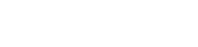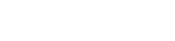# GMAT Tricks with Systems of Equations: Part 5

A lot of GMAT test-takers vaguely remember a rule from high school, that it’s possible to solve for two variables if and only if you’re given two equations, and generally that it’s possible to solve for n variables if and only if you’re given n equations. Unfortunately, that rule isn’t quite correct as written, and even the correct rule isn’t always relevant.

Applying the rule incorrectly causes quite a few errors on the quant section, particularly with Data Sufficiency questions. This is the fifth in a series of posts on avoiding those errors:

In my first three posts on this topic we saw that sometimes two equations aren’t enough to allow us to solve for two variables, or even for one. That is, we saw that sometimes, information that seems sufficient isn’t in fact sufficient.

The trick was that the rule above isn’t correct as written. The correct rule is that a system of n distinct linear equation is sufficient to solve for n variables, but that sometimes the GMAT gives you systems of equivalent (not distinct) equations, or exponential or quadratic (not linear) equations, and that the rule doesn’t apply to such systems.

In my last post we saw that sometimes information that doesn’t seem sufficient turns out to be, even when the equations in question are distinct and linear, because the story problem imposes implicit constraints that the equations might miss.

In this post we’ll see another sort of system of equations for which information that doesn’t seem sufficient turns out to be.

## A Sample Problem

Take a couple of minutes to tackle this problem:

If 4x – z = 6y + z, what is the value of z?

(1) 2x – 5 = 3y
(2) 3x – 11 = 2z

(A) Statement (1) ALONE is sufficient, but statement (2) alone is not sufficient.
(B) Statement (2) ALONE is sufficient, but statement (1) alone is not sufficient.
(C) BOTH statements TOGETHER are sufficient, but NEITHER statement ALONE is sufficient.
(D) EACH statement ALONE is sufficient.
(E) Statements (1) and (2) TOGETHER are NOT sufficient.

In this case, if we too quickly apply the rule described above, we’ll probably choose (C). After all, we have three variables—x, y, and z—and so presumably we need three equations, the one in the question stem and the two in the statements.

And, though the correct answer isn’t in fact (C), there’s something to that logic. We would in fact need all three equations to determine the values of all three variables, but our question asks just for the value of z.

## What Is This Question Really Asking?

Whenever the question stem presents you with an equation with multiple variables and asks you to solve for one of them, you should immediately rewrite the equation to find a more useful question.

Specifically, you should isolate the variable you’re asked to solve for and then focus on the expression equal to that variable. Here’s what that would look like for this problem:

4x – z = 6y + z

Add z to each side of the equation and subtract 6y from each side.

2z = 4x – 6y

Divide each side by 2.

z = 2x – 3y

So our real question is, “What is the value of 2x – 3y?”

## Now Turn to Statement (1)

Does the equation 2x – 5 = 3y allow us to solve for 2x – 3y?

Manipulate the left-hand side of the equation to isolate 2x – 3y and let the right-hand side of the equation take care of itself.

2x – 5 = 3y

Subtract 3y to each side of the equation and add 5 to each side.

2x -3y = 5

So Statement (1) is sufficient. Eliminate answers (B), (C), and (E).

## Now Turn to Statement (2)

Does the equation 3x – 11 = 2z allow us to solve for 2x – 3y?

Perhaps you can see right away that it does not, since that equation incudes the variable z (which we don’t want) but doesn’t include the variable y (which we need). If that isn’t obvious, proceed as above: manipulate the left-hand side of the equation to isolate 2x – 3y and let the right-hand side of the equation take care of itself.

3x – 11 = 2z

3x = 2z + 11

Multiply each side by 2/3.Subtract 3y from each side.Since the right-hand side of the equation is not a constant, it doesn’t appear as though Statement (2) is sufficient by itself. Eliminate (D). The correct answer is (A).

## Author

•Michael Schwartz is really good at standardized tests. He’s earned multiple perfect scores on the GRE, GMAT, and LSAT. He’d rather have perfect pitch or be able to run low 1:40s for the 800 meters, but you take what you get. He has decades of teaching and curriculum-development experience. One of these days he might finish his dissertation and collect that Ph.D. in philosophy. Might.